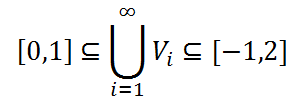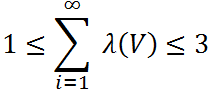Thursday, March 14, 2013

Lebesgue Measure III

For an introduction to the Lebesgue measure and various applications to sets in Rn, see the previous two posts, beginning here.

The utility of the Lebesgue Measure has been described in the previous two posts. To briefly summarize, the Lebesgue measure provides a more general notion of the "size" of a set in space that matches up with the intuitive notions of length, area and volume in 1, 2, and 3 dimensions, respectively, as well as preserving natural properties such as the volume of a union of disjoint sets being the sum of volumes of the individual sets. It even goes beyond these notions to measure countable and uncountable sets, manifolds, and even fractals of various sorts. However, under certain assumptions, there exist sets which cannot be consistently assigned a Lebesgue Measure. Such sets and the validity of the underlying assumption necessary for their existence are discussed in this post.

For the construction of these Lebesgue non-measurable sets or Vitali Sets, one must assume one of the most dubious and controversial statements in mathematics: the axiom of choice.

The axiom of choice is not by any means specific to measure theory, but rather is an axiom of set theory, and therefore lies at the foundation of mathematics. The axiom, put informally, is the seemingly innocent statement below:

For any family of sets, there exists a way of choosing (hence the name "axiom of choice") one member from each set in the family.

Slightly more formally, it states that for any set X containing only nonempty sets as members, there exists a choice function f which selects exactly one member from each member of X. Of course, there may be many choice functions for a collection X of sets; the axiom simply guarantees that at least one exists. For example, set
X = {x1,x2,x3} = {{4,5,6},{1,4,7},{2,7,9}}. A choice function on this collection of sets maps each subset of X to one of its members. For example, one could have
f(x1) = f({4,5,6}) = 4, and the function would be similarly defined on x2 and x3.

The act of selecting one member from each of a class of sets seems completely natural and perhaps even fundamental. It seems impossible to imagine a collection of sets where such a choice function would not be possible. Yet the axiom of choice implies the existence of a set that is not measurable by the Lebesgue Measure, and therefore has no definable volume. That such a set exists and can be embedded in a "well-behaved" space like Rn seems surprising, almost contradictory. Without further ado, let us construct a Vitali Set.

For simplicity, the Vitali Set considered will be in R1. The first step in constructing a Vitali Set relies on a concept called a quotient group, specifically the quotient of the real numbers and the rational numbers, denoted R/Q.

R/Q contains a number of classes. Each class is the set of rational numbers Q shifted by a real number r. More specifically, each class, denoted Q + r, contains every number that is formed by adding a rational number to r. For example, if r is π, a real number, then Q + π contains members such as 3/4 + π and -9 + π, but not multiples of π such as π/2, nor rational numbers themselves (e.g. 1/2). Conversely, a real number x is a member of Q + r if and only if x - r is rational. Finally, to form the quotient group R/Q, we discard any "repeats", so that each pair of classes Q + r and Q + s in R/Q is disjoint, i.e. the two have no common elements.

Since the real numbers form an uncountable set, but each class Q + r has as many members as the rational numbers and is countable, the quotient group R/Q is an uncountable set, containing countable sets as members. It is also a partition of R, as every real number is contained within one of the classes, but only one, as they are disjoint.

The next step in construction makes use of the axiom of choice. Clearly, each class
Q + r contains members in the interval [0,1] (as each is dense in the real numbers). Therefore, for each class Q + r, the set (Q + r)∩([0,1]) (∩ denotes the intersection of two sets) is nonempty. The axiom of choice then allows one to select exactly one member from each (Q + r)∩([0,1]), and combine them into their own set. The resulting set has one member for each class of R/Q, all within the interval [0,1].

Call the set just formed V. Note that V was not determined in any precise sense, and rather could be any one of an infinity of possible sets satisfying the same conditions, but for a different selection of choice functions. Furthermore, V is uncountable, and for every real number r, there is exactly one vV such that v - r is rational. Also, taking r = 0, there is exactly one rational number in V.

V is the Vitali set. Now it must be proven that it does not have a Lebesgue measure. The final step involves translations of V itself, formed in a manner analogous to those of the classes of R/Q. This time, however, the shifts will be of certain rational numbers.

Since the rational numbers are countable, we can make use of a "catalog" of them, indexed by the positive integers. In other words, we can list the rational numbers, and assign every one a number which will serve as its index. This is called an enumeration of the rational numbers. The enumeration, in this case, will be confined to the rational numbers in the interval [-1,1]. As with the selection of choice functions, the enumeration of these numbers chosen is arbitrary, as differences between specific enumerations will not affect the final result. Denote each rational number in the list qi, for a positive integer i. For example, one could have q1 = 0, q2 = 1/2, q3 = -2/5, etc..

Now we form, for each qi, the set Vi = V + qi, or the set of all numbers created by adding qi to a member of V. It is very important to note that the resulting sets are pairwise disjoint; no two of the translations contain a common member. To see this, remember that each member v of V is representative of a class of the form Q + r. There is one, and only one member of V for every class of the above form in R/Q. The corresponding member in each Vi will also be a member of the class Q + r, as it differs from v only by a rational number. Therefore, it cannot be the same as any member of any Vi formed from another class Q + s. Finally, since the qi are distinct, no two translations of the member v of the original V yield the same number.

After this fact is accepted, the non-measurability of V can be proven. The set that must next be considered is actually the union of of all the Vi. Since there are countably many Vi, and they are pairwise disjoint, the Lebesgue measure of the union is the sum of the Lebesgue measures of each Vi. Hence,What is the value of this sum? We can place some limitations on it by an examination of the union of the Vi. Note first that every real number on the interval [0,1] is contained within the union of the Vi, since the original V contains representative members of each class in a partition of R/Q. Since these representative elements are within [0,1], it is only necessary to shift them by a number such that |q| ≤ 1, because a distance of 1 is the "farthest away" two objects in [0,1] can be from one another. This reveals the purpose of the enumeration only being of the rational numbers between -1 and 1. Shifting a single element v of V that represented the class Q + r by all of the qi (which lie in the interval [-1,1]) will recreate all of Q + r in [0,1], as well as more outside the interval.

The logical basis for the second limitation is even simpler. Since each v in V must be within [0,1], and the maximum shift induced by the qi to form the Vi has a magnitude of 1, all members of the union of the Vi must be within [-1,2]. More precisely, the smallest possible member of V is 0, causing the smallest possible member of the Vi to be -1, and the largest possible member of V is 1, causing the largest possible member of the Vi to be 2. The above limitations are illustrated in equation below:We can now use the simple property of the Lebesgue measure that if a set A is contained within another set B, then λ(A) ≤ λ(B). Substituting inequality for inclusion, we can then replace the sets in the above equation with their measures:Note, finally, that a translation of a set has the same measure as the original set. This is a basic property of the Lebesgue measure, and is reflected in one's intuitive notion of Euclidean geometry; moving figures around in the plane, for example, does not change their area. In light of this property, since each Vi is a translation of V, the last inequality becomesHowever, this is a contradiction. Since the expression in the infinite sum is independent of i, it is simply a constant added to itself an infinite number of times. However, the Lebesgue measure of a set can only be a positive real number or zero, and neither of these, when added to itself an infinite number of times, can produce a number between 1 and 3. The assumption that V is measurable is therefore false.

V is not unique of course, due to the different choice function possibilities. In fact, uncountably many Vitali sets arise from the above construction. The idea that so many sets on such a simple structure as the real number line could be non-measurable is remarkable. This brings us back to the main question: is the axiom of choice valid?

There is little consensus on this topic among mathematicians. The axiom itself (in its set-theoretical form) seems intuitive but there are many equivalent or implied statements that seem counterintuitive, such as the result above. Non-measurable sets have been applied to formulate a statement that seems so blatantly contradictory that it is called the Banach-Tarski paradox. It states that, given the axiom of choice, one can subdivide a three-dimensional ball into discrete pieces, and, without altering them, rearrange them in such a way that two three-dimensional balls are formed of identical size to the first. Such an act of "cloning" in reality is contrary to common physical principles. Yet mathematically, many important and seemingly valid theorems depend on the axiom. Therefore, there is evidence for both arguments, and the matter is not likely to be conclusively resolved in the near future.

Returning briefly to the Lebesgue measure, the effectiveness and generality of this instrument, despite the above defect, has led to growth in many related areas of mathematics. Lebesgue himself used the measure and related concepts to more rigorously define properties of functions on Rn, including those which, in earlier periods, were often disregarded. Since the theory of measure is related to that of manifolds, the above developments had great significance in the area of topology as well.

Sources: Vitali Sets, Axiom of Choice on Wikipedia, http://homepage.univie.ac.at/erhard.reschenhofer/pdf/probstat/P_A.pdf